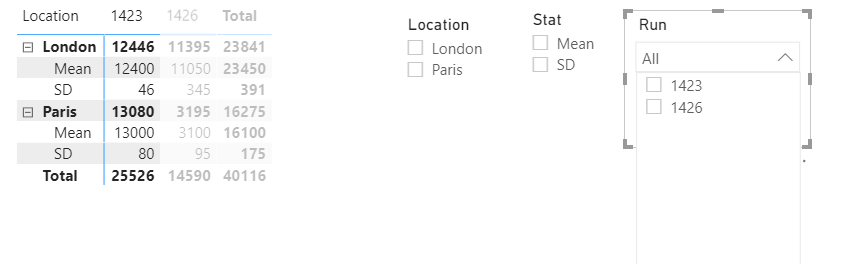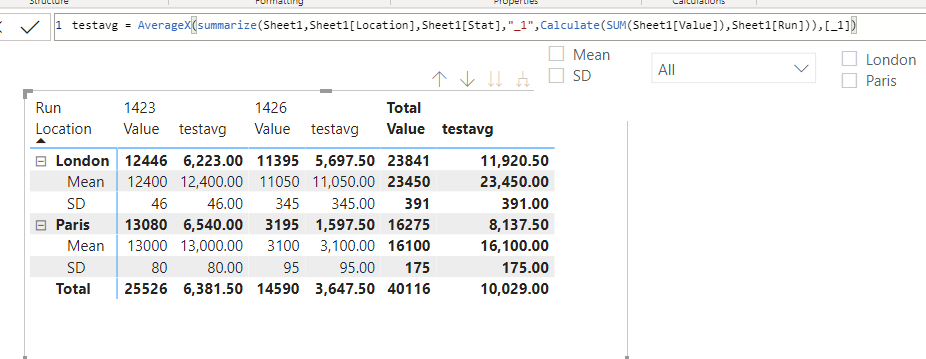cancel
Showing results for
Did you mean:Helper I

## Sum over filtered values and replace total with average

Hello all!

I have found lots of similar answers to similar questions on the forums already, but none seem to work so hoping someone could help me out.

I have the following matrix visualisation which I use drop down menus to filter.I wish to compare the different runs, which make up my column variable, and then filter those by my other variables for more detail. I would mainly like my total column to be replaced by an averages column and then have additional columns side by side with the differences between that run and the average.

I understand the basics of measures and have had plenty of attempts at making my own average column, but when I use DAX like:

SumOverRun = Calculate(SUM(TestData[Value]),TestData[Run])
It sums over all runs, even when I have used the dropdowns to filter away some of them.
Once I have figured out how to sum over just the filtered values it seems relatively simple to calculate the average using countrows(distinct)
, and then subtracting the values away from that, before using conditional formatting to colour these differences, but if anyone has insight on issues I may face,
that would be appreciated too!

Thanks,
EHa

1 ACCEPTED SOLUTIONHelper I

Going to drop my solution in here ion case anyone with a similar problem comes across it in the future:

For me it was as simple as :

TotalSum  = Sum(TableName[Value])

RunsSelected = Countrows(Distinct(AllSELECTED(TableName[Runs])))

SumOverRuns = Calculate( [TotalSum] , ALLSELECTED(TableName[Runs]))

AverageOverRuns = Divide(SumOverRuns , RunsSelected)

3 REPLIES 3Super User

@EHa , is filtered or is in scope should help

https://powerpivotpro.com/2013/03/hasonevalue-vs-isfiltered-vs-hasonefilter/

or see this formula can help

``averageX(summarize(table,table[location], table[stat],"_1",Calculate(SUM(TestData[Value]),TestData[Run])) ,[_1])``

!! Microsoft Fabric !!
Microsoft Power BI Learning Resources, 2023 !!
Learn Power BI - Full Course with Dec-2022, with Window, Index, Offset, 100+ Topics !!
Did I answer your question? Mark my post as a solution! Appreciate your Kudos !! Proud to be a Super User! !!Helper I

Hi @amitchandak ,

Thanks for the quick reply , I will definitely have a proper explore of the isfiltered function today.

I did try your test code (with both table and testdata changed to Sheet1 ,which is the name of the table in my report at the moment)

It doesn't seem to do what I want however:Idon't fully understand what it is doing in every position, but it seems to do exactly what I want for London and Paris totals, but not for the Mean and SD as it is dividing the totals by 1 and not 2. Is there a way to slightly edit this so it works do you think?

Additionally, once this is done, is there a way to only keep the final column / turn the others to differences?

Thanks a lot for your help,

EHaHelper I

Going to drop my solution in here ion case anyone with a similar problem comes across it in the future:

For me it was as simple as :

TotalSum  = Sum(TableName[Value])

RunsSelected = Countrows(Distinct(AllSELECTED(TableName[Runs])))

SumOverRuns = Calculate( [TotalSum] , ALLSELECTED(TableName[Runs]))

AverageOverRuns = Divide(SumOverRuns , RunsSelected)